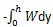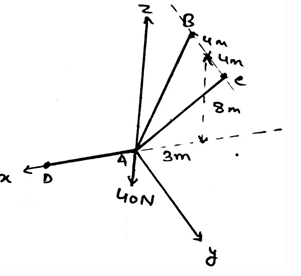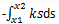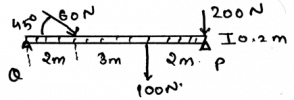Test: Conservative Forces

# Test: Conservative Forces

Test Description

## 15 Questions MCQ Test Engineering Mechanics | Test: Conservative Forces

Test: Conservative Forces for Mechanical Engineering 2023 is part of Engineering Mechanics preparation. The Test: Conservative Forces questions and answers have been prepared according to the Mechanical Engineering exam syllabus.The Test: Conservative Forces MCQs are made for Mechanical Engineering 2023 Exam. Find important definitions, questions, notes, meanings, examples, exercises, MCQs and online tests for Test: Conservative Forces below.
Solutions of Test: Conservative Forces questions in English are available as part of our Engineering Mechanics for Mechanical Engineering & Test: Conservative Forces solutions in Hindi for Engineering Mechanics course. Download more important topics, notes, lectures and mock test series for Mechanical Engineering Exam by signing up for free. Attempt Test: Conservative Forces | 15 questions in 30 minutes | Mock test for Mechanical Engineering preparation | Free important questions MCQ to study Engineering Mechanics for Mechanical Engineering Exam | Download free PDF with solutions
 1 Crore+ students have signed up on EduRev. Have you?
Test: Conservative Forces - Question 1

### If a block moves from a height h above the ground then the work done is given by_______

Detailed Solution for Test: Conservative Forces - Question 1

The potential energy is converted into the work done. Thus is the block moves from the height h to the ground, the work done is given by.

Negative because the work done is in the opposite direction of the motion of the body.

Test: Conservative Forces - Question 2

### If any external conservative force also is applied on the distributed loading then?

Detailed Solution for Test: Conservative Forces - Question 2

The external forces are treated differently. They are not added by the force of the distributed loading. That is the force not only acts at the centroid always. It can be shifted also. Depending on the external forces. Thus the use of centroid or centre of mass.

Test: Conservative Forces - Question 3

### Find the tension in the cable AC.Detailed Solution for Test: Conservative Forces - Question 3

First represent the forces in their vector form. Then equate the net sum of the forces in the x, y and z directions to be zero. You will get FB = FC and 2(.848) = 40N. This gives the answer as 23.6N.

Test: Conservative Forces - Question 4

What is the sum of squares of the cosine angles made by the conservative force vector with the coordinate axis?

Detailed Solution for Test: Conservative Forces - Question 4

The sum of the squares of the cosines of the vector will give you the squares of the components in the numerator, and the vector’s magnitude’s square in the denominator. But the numerator sum is equal to the vector’s magnitude’s square. Thus, the answer = 1.

Test: Conservative Forces - Question 5

____________ is the phenomena that resist the movement of the two surfaces in contact. This phenomena is also having its scope in the work and the conservative nature of the force.

Detailed Solution for Test: Conservative Forces - Question 5

The friction is the phenomena that defines that there is a resistance which is present there between the two surfaces. The two surfaces are in contact and the friction applies at that surface only, resisting the motion of the surface.

Test: Conservative Forces - Question 6

In the explanation of the theory of friction over which conservative forces were acted upon, the block used have an assumption. That is the upper portion of the block is considered to be rigid.

Detailed Solution for Test: Conservative Forces - Question 6

The block used to explain the theory of friction is placed on a deformable surface. As we know that the theory of friction says that when there is no lubricating fluid present between the surfaces in contact, the dry friction occurs. Thus to show the same, experiments are done over a non-rigid/deformable surface which is having a rigid shape.

Test: Conservative Forces - Question 7

Work done by a spring to a body from moving it from x1 to x2 distance is given by _________

Detailed Solution for Test: Conservative Forces - Question 7

The potential energy is converted into the work done. Thus is the block moves from the distance x2 to x1, the work done is given by. Negative because the work done is in the opposite direction of the motion of the body.

Test: Conservative Forces - Question 8

Which statement is right for conservative force vector F = Ai + Bj + Ck?

Detailed Solution for Test: Conservative Forces - Question 8

As given the vector is F = Ai + Bj + Ck, this implies that the x ,y and z-axis components of this vector is A, B and C respectively. But, in rectangular components representation of any vector, the vector is written as F = Fx + Fy + Fz.

Test: Conservative Forces - Question 9

For equilibrium the net moment acting on the body by various conservative forces is zero.

Detailed Solution for Test: Conservative Forces - Question 9

The equilibrium is only attained if the net moment on the body tends to be equal to zero. Thus the moments caused by different forces cancels out. If this happens there is no motion of the body along any direction and hence the body is said to be in equilibrium. The body here is a rigid body.

Test: Conservative Forces - Question 10

Determine the horizontal components of the reaction on the beam caused by the pin at Q. The force 60N is multiplied by 10 and then is applied.Detailed Solution for Test: Conservative Forces - Question 10

The summation of the forces needs to be zero. So does the summation of the moments need to zero. But talking about the angles, they not needed to zero. But the forces which are acting at particular angles, must needed to.

Test: Conservative Forces - Question 11

The conservative frictional force always acts ____________ to the surface of the application of the friction.

Detailed Solution for Test: Conservative Forces - Question 11

The friction is the phenomena that defines that there is a resistance which is present there between the two surfaces. This friction is applied tangentially to the surfaces in contact. Thus the main thing is that the forces on both of the surfaces act tangential to each other.

Test: Conservative Forces - Question 12

What does the Newton’s third law states for the conservative forces?

Detailed Solution for Test: Conservative Forces - Question 12

The requirement of the third law is important in the equilibrium of the body. Specially the rigid bodies. The rigid body particles are in the equilibrium and are thus facing the forces and to be in the equilibrium they also react and apply the opposite force and thus the third law of newton.It is same for all the methods.

Test: Conservative Forces - Question 13

We use sometimes the measures to know the direction of moment in the calculations of the moments caused by the conservative forces. Which one is right about it(consider the mentioned axis to be positive)?

Detailed Solution for Test: Conservative Forces - Question 13

As right handed coordinate system means that you are curling your fingers from positive x-axis towards y-axis and the thumb which is projected is pointed to the positive z-axis. Thus visualizing the same and knowing the basic members of axis will not create much problem.

Test: Conservative Forces - Question 14

For the conditions of the equilibrium of the body, i.e. the rigid body only the external conservative forces defines the equilibrium. Because the internal conservative forces cancels out so not to be considered.

Detailed Solution for Test: Conservative Forces - Question 14

The application of the internal forces does affect the conditions of the equilibrium of the body. Not only the external but the internal forces that are developed by the sake of external forces does develop a tending effect on the equilibrium of the body. Thus the internal forces doesn’t cancels out.

Test: Conservative Forces - Question 15

Which statement is correct about the vector F in the showing the conservative nature of the forces?

Detailed Solution for Test: Conservative Forces - Question 15

As we know the α, β and γ are the angles made by the x, y and z-axis respectively. Thus, is the magnitude of the vector is F, the F= Fcos β + Fcos α + Fcosγ. Which means the force is the resultant of all its axis’ components.

## Engineering Mechanics

24 videos|71 docs|58 tests
 Use Code STAYHOME200 and get INR 200 additional OFF Use Coupon Code
Information about Test: Conservative Forces Page
In this test you can find the Exam questions for Test: Conservative Forces solved & explained in the simplest way possible. Besides giving Questions and answers for Test: Conservative Forces, EduRev gives you an ample number of Online tests for practice

## Engineering Mechanics

24 videos|71 docs|58 tests